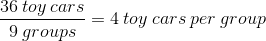SSAT Elementary Level Math : How to divide

Example Questions

Example Question #11 : How To Divide

Which of the numbers is not prime?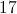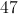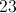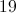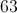Explanation:

A prime number is only divisible by itslef and. This is true of all the given numbers except for, which is divisible by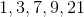andExample Question #12 : How To Divide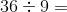Explanation:

The correct answer is 4.  The question is asking how many times 9 goes into 36.  Think 9, 18, 27, and then 36, so 4 times.

Example Question #13 : How To Divide

Find the quotient and show a remainder if necessary. Remainders are expressed as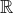.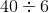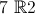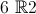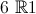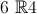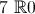Explanation:

The correct answer is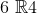.  6 goes into forty 6 times (36).  Because 36 is four away from 40, the remainder is 4.

Example Question #2 : Fluently Add, Subtract, Multiply, And Divide Multi Digit Decimals: Ccss.Math.Content.6.Ns.B.3

Solve for: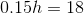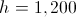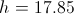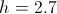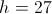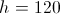Explanation: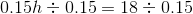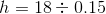To divide, move the decimal point in both numbers right two places to make the divisor whole.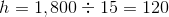Example Question #14 : How To Divide

Tarleton has 105 marbles. He decides to break his marbles up into 5 equal groups. How many marbles will be in each group?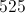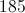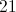Explanation:

Because we are breaking the total number of marbles into smaller, equal groups, we need to divide!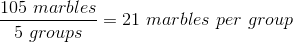Example Question #15 : How To Divide

John arranges 20 toy cars into 5 equal groups. How many toy cars does John have in each group?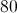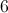Explanation:

Since John is breaking down his total number of cars into smaller, equal groups, we need to divide!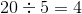Example Question #16 : How To Divide

Jenny has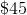to spend on food for the nextdays. If she spends her entire budget by spending the same amount each day, how much does she spend each day?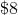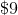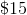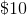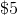Explanation:

To find out how much ofwould be spent per day overdays, dividebydays.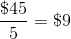per day

Example Question #17 : How To Divide

Dan hastokens. If he shares his tokens evenly between himself and three friends, how many extra tokens will be left over?tokenstokenstokenstokenstokentokens

Explanation:

Divide the total number of tokens by the total number of people, then find the remainder to see how many tokens will be left over.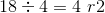Each person will receivetokens, with a remainder oftokens.

Example Question #18 : How To Divide

Tyler started his hike with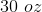ounces of water in his water bottle. At the end of his hike, he has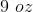remaining.

If Tyler hiked forhours, how many ounces of water did Tyler drink per hour?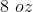per hour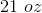per hour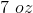per hour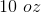per hour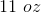per hourper hour

Explanation:

Subtract the amount of water Tyler had remaining from the amount he began with to find out how much he drank.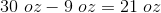Divide the amount he drank by the number of hours he hiked to find out how much water he drank per hour.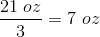per hour

Example Question #19 : How To Divide

James has 36 toy cars. If James decides to break his toy cars into 9 equal groups, how many toy cars will be in each group?